Your browser does not support the IFRAME tag.Home MonkeyNotes Printable Notes Digital Library Study Guides Study Smart Parents Tips College Planning Test Prep Fun Zone Help / FAQ How to Cite New Title Request

 8.4 Mean Value If y = f (x) is given a function defined on [a, b], then the mean value of the function denoted by y = f (c) is defined as y or f (c) =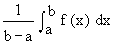for some c Î (a, b) Example 27 Find the mean value of y =over [0, 2] Solution : By Mean value theorem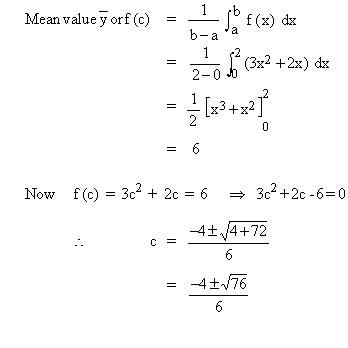The mean value theorem is also satisfied. Example 28 Given that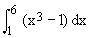= 318 Find the mean value of x3 - 1 and also find all 'c' values that satisfies the mean value theorem for this function on the closed interval. Solution :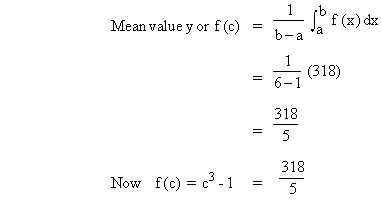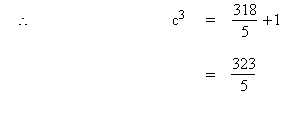\ c » 4 is in the closed interval (1, 6) \ The mean-value theorem is satisfied. Index Chapter 1